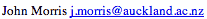Data Structures and Algorithms 9.2 Binomial Coefficients

As with the Fibonacci numbers, the binomial coefficients can be calculated recursively - making use of the relation:

nCm = n-1Cm-1 + n-1Cm
A similar analysis to that used for the Fibonacci numbers shows that the time complexity using this approach is also the binomial coefficient itself.

However, we all know that if we construct Pascal's triangle, the nth row gives all the values,
nCm, m = 0,n:

 ``` 1 1 1 1 2 1 1 3 3 1 1 4 6 4 1 1 5 10 10 5 1 1 6 15 20 15 6 1 1 7 21 35 35 21 7 1 ```
Each entry takes O(1) time to calculate and there are O(n2) of them. So this calculation of the coefficients takes O(n2) time. But it uses O(n2) space to store the coefficients.

©, 1998